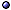Types of Geodesics

In General > see geodesics [quantum corrections]; projective structures.
* Homogeneous geodesics: In any homogeneous (Riemannian or pseudo-Riemannian) manifold, there is at least one homogeneous geodesic through each point.
* Compact Lorentzian manifolds: All lightlike geodesics are periodic, while there are closed and non-closed spacelike and timelike geodesics.
@ Homogeneous geodesics: Dusek JGP(10).
@ Conformal geodesics: Friedrich & Schmidt PRS(87); Friedrich CMP(03)gq/02; Tod JGP(12) [examples].
@ Riemannian manifolds: Berger 65; Bolsinov & Jovanović in(04)mp/03 [integrability]; LaGatta & Wehr CMP(14)-a1206, a1206 [random perturbations of Euclidean space].
@ Lorentzian manifolds: Larsen JDG(96) [sic]; Candela & Sánchez in(08)m.DG/06 [rev]; Bolsinov et al JLMS(09)-a0806 [Fubini theorem, for pseudo-Riemannian metrics]; Del Barco et al JGP(14) [compact Lorentzian manifolds]; > s.a. finsler geometry.
@ 2D: Knieper & Weiss JDG(94) [S2, positive topological entropy]; Lévay JPA(00), JPA(00) [negative curvature Riemann surfaces]; Rowland EJP(06) [simple]; Ying & Candès JCP(06) [computation]; Müller PLA(12) [on closed surfaces, numerical, and chaotic regions].
@ 3D: Bose et al CG(12) [3D polyhedral surfaces].
> Related topics: see Congruence and Congruence Expansion; Totally Geodesic [mapping, submanifold].

Null Geodesics > s.a. coordinates [based on a null geodesic]; quantum spacetime; spacetime subsets [and complex world-lines].
* Set of null geodesics: If $$(M,g)$$ is a globally hyperbolic (d+1)-dimensional spacetime, this set $$\cal N$$ is naturally a smooth $$(2d-1)$$-dimensional contact manifold.
* Sky: The sky of an event is the subset of $$\cal N$$ defined by all null geodesics through that event, and is an embedded Legendrian submanifold of $$\cal N$$ diffeomorphic to a S$$^{d-1}$$.
@ General references: Low JMP(89); Urbantke JMP(89); Frittelli & Newman gq/98-fs [time of arrival].
@ Types of spacetimes: Frittelli et al CQG(98) [asymptotically flat, conjugate points]; Hall & Lonie JMP(08) [FLRW spacetimes]; Décanini et al PRD(10)-a1002 [circular, static spherically symmetric black holes]; Gibbons & Vyska CQG(12)-a1110 [spherically symmetric, and Weierstraß elliptic functions]; Bikwa et al MNRAS(12)-a1112 [FLRW spacetimes]; Kraniotis GRG(14)-a1401 [Kerr-Newman (-de Sitter) black holes]; Paganini et al a1611 [Kerr]; Charbulák & Stuchlík EPJC(17)-a1702 [Kerr-de Sitter]; Gal'tsov & Kobialko PRD(19)-a1901 [Kerr and Kerr-Newman].
@ Null geodesic congruences: Adamo et al LRR(09)-a0906, LRR(12) [asymptotically shear-free]; Adamo & Newman CQG(11) [generalized good cut equation]; Newman GRG(20) [in Minkowski spacetime].
@ Properties of skies: Natário CQG(02)gq/01 [skies]; Natário & Tod PLMS(04)gq/02; Bautista et al CQG(15)-a1411 [partial order on the space of skies, and the Malament-Hawking theorem].

Special Types of Spacetimes > s.a. connections [non-commutative]; graph theory; regge calculus; types of lorentzian metrics.
* Stationary axisymmetric spacetimes: The motion of a test particle in a stationary axisymmetric gravitational field is generally non-integrable unless, in addition to the energy and angular momentum about the symmetry axis, an extra non-trivial constant of motion exists, as in the Kerr spacetime.
@ Stationary axisymmetric spacetimes: Brink PRD(08)-a0807 [vacuum]; Markakis MNRAS(14)-a1202 [constants of the motion]; Pineda et al a1409-proc; > s.a. solutions with symmetries [static].
@ Other black-hole spacetimes: Marck CQG(96)gq/95 [Schwarzschild-Kerr]; Cardoso et al PRD(09)-a0812 [stability, Lyapunov exponents, and quasinormal modes]; Slany et al IJMPA(09) [Kerr-de Sitter]; Radosz et al MPLA(11) [black holes in Hořava-Lifshitz gravity]; Chakraborty & Chakraborty CJP(11)-a1109 [spherically symmetric, test-particle motion and light bending]; Yang & Wang A&A(14)-a1311 [Kerr-Newman spacetime, numerical code ynogkm for timelike geodesics]; Pradhan CQG(15)-a1402 [Kerr-Newman-Taub-Nut spacetime]; Hackmann a1506-proc, Hackmann & Lämmerzahl AIP(14)-a1506 [Plebański-Demiański family of solutions]; Semerák & Suková in(15)-a1509 [around deformed black holes]; Salazar & Zannias PRD(17)-a1705 [Kerr-de Sitter]; Liu et al CQG(17)-a1706 [Kerr-Newman spacetime, neutral particles]; Eskin RVMP(19)-a1807 [Reissner-Nordström spacetime]; > s.a. spinning particles.
@ Bianchi metrics: Nilsson et al GRG(00)gq/99 = GRG(00) [dynamical system]; Valent & Yahia CQG(07) [integrable flows]; > s.a. bianchi models.
@ Cosmological metrics: Barrow & Levin PLA(97) [k = −1 FLRW models]; Podolský & Belán CQG(04) [Kundt spacetimes]; Pereira & Sampson GRG(12)-a1110 [de Sitter spacetime, generalized geodesics]; Sokołowski & Golda IJMPD(16)-a1602 [in AdS spacetime].
@ Other metrics: Moraes PLA(96) [dislocation]; Herrera FPL(05) [quash-spherical]; D'Afonseca et al CQG(05)gq [Weyl-Bach ring solution]; Kiosak & Matveev CMP(09)-a0806 [geodesically rigid]; Andrade & de Berredo-Peixoto G&C(13)-a1203 [spherically symmetric dislocation]; Lim PRD(14)-a1405 [vacuum C-metric]; Podolský et al CQG(15)-a1409 [non-expanding impulsive gravitational waves]; Bambhaniya et al PRD(19)-a1908 [with naked singularities].
@ Generalized settings: Ulhoa et al GRG(14)-a1312 [non-commutative spacetime]; Arrighi & Dowek a1507 [discrete spacetime]; > s.a. finsler geometry [Randers spaces]; geodesics.Black-hole metrics: see chaotic motion; particles in kerr spacetimes; particles in schwarzschild spacetimes; reissner-nordström spacetimes.Other metrics: see C-metric; FLRW metrics; gödel solution; gowdy spacetime; Lemaître-Tolman-Bondi Solution; Lewis Metric; multipole moments.